Courses

# Test: Arithmetic Reasoning- 2

## 20 Questions MCQ Test Logical Reasoning (LR) and Data Interpretation (DI) | Test: Arithmetic Reasoning- 2

Description
This mock test of Test: Arithmetic Reasoning- 2 for LR helps you for every LR entrance exam. This contains 20 Multiple Choice Questions for LR Test: Arithmetic Reasoning- 2 (mcq) to study with solutions a complete question bank. The solved questions answers in this Test: Arithmetic Reasoning- 2 quiz give you a good mix of easy questions and tough questions. LR students definitely take this Test: Arithmetic Reasoning- 2 exercise for a better result in the exam. You can find other Test: Arithmetic Reasoning- 2 extra questions, long questions & short questions for LR on EduRev as well by searching above.
QUESTION: 1

### Five bells begin to toll together and toll respectively at intervals of 6, 5, 7, 10 and 12 seconds. How many times will they toll together in one hour excluding the one at the start ?

Solution:

L.C.M. of 6, 5, 7, 10 and 12 is 420.

So, the bells will toll together after every 420 seconds i.e. 7 minutes.

Now, 7 x 8 = 56 and 7 x 9 = 63.

Thus, in 1-hour (or 60 minutes), the bells will toll together 8 times, excluding the one at the start.

QUESTION: 2

### A bus starts from city X. The number of women in the bus is half of the number of men. In city Y, 10 men leave the bus and five women enter. Now, number of men and women is equal. In the beginning, how many passengers entered the bus ?

Solution:

Originally, let number of women = x. Then, number of men = 2x.

So, in city Y, we have : (2x - 10) = (x + 5) or x - 15.

Therefore Total number of passengers in the beginning = (x + 2x) = 3x = 45.

QUESTION: 3

### A, B, C, D and E play a game of cards. A says to B, "If you give me 3 cards, you will have as many as I have at this moment while if D takes 5 cards from you, he will have as many as E has." A and C together have twice as many cards as E has. B and D together also have the same number of cards as A and C taken together. If together they have 150 cards, how many cards has C got ?

Solution:

Clearly, we have :

A = B - 3 ...(i)

D + 5 = E ...(ii)

A+C = 2E ...(iii)

B + D = A+C = 2E ...(iv)

A+B + C + D + E=150 ...(v)

From (iii), (iv) and (v), we get: 5E = 150 or E = 30.

Putting E = 30 in (ii), we get: D = 25.

Putting E = 30 and D = 25 in (iv), we get: B = 35.

Putting B = 35 in (i), we get: A = 32.

Putting A = 32 and E = 30 in (iii), we get: C = 28.

QUESTION: 4

A farmer built a fence around his square plot. He used 27 fence poles on each side of the square. How many poles did he need altogether ?

Solution:

Since each pole at the corner of the plot is common to its two sides, so we have :

Total number of poles needed = 27 x 4 - 4 = 108 - 4 = 104.

QUESTION: 5

In a city, 40% of the adults are illiterate while 85% of the children are literate. If the ratio of the adults to that of the children is 2 : 3, then what percent of the population is literate ?

Solution: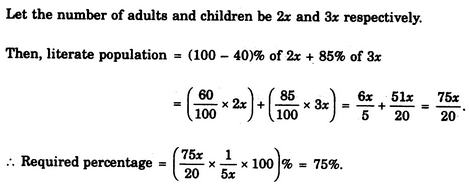QUESTION: 6

A is three times as old as B. C was twice-as old as A four years ago. In four years' time, A will be 31. What are the present ages of B and C ?

Solution:

We have : A = 3B ...(i) and

C - 4 = 2 (A - 4) ...(ii)

Also, A + 4 = 31 or A= 31-4 = 27.

Putting A = 27 in (i), we get: B = 9.

Putting A = 27 in (ii), we get C = 50.

QUESTION: 7

Today is Varun's birthday. One year, from today he will be twice as old as he was 12 years ago. How old is Varun today ?

Solution:

Let Varan's age today = x years.

Then, Varan's age after 1 year = (x + 1) years.

Therefore x + 1 = 2 (x - 12)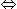x + 1 = 2x - 24x = 25.

QUESTION: 8

A bird shooter was askgd how many birds he had in the bag. He replied that there were all sparrows but six, all pigeons but six, and all ducks but six. How many birds he had in the bag in all?

Solution:

There were all sparrows but six' means that six birds were not sparrows but only pigeons and ducks.

Similarly, number of sparrows + number of ducks = 6 and number of sparrows + number of pigeons = 6.

This is possible when there are 3 sparrows, 3 pigeons and 3 ducks i.e. 9 birds in all.

QUESTION: 9

Mr. Johnson was to earn � 300 and a free holiday for seven weeks' work. He worked for only 4 weeks and earned � 30 and a free holiday. What was the value of the holiday?

Solution: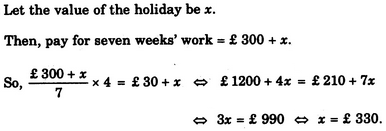QUESTION: 10

What is the smallest number of ducks that could swim in this formation - two ducks in front of a duck, two ducks behind a duck and a duck between two ducks ?

Solution:

Clearly, the smallest such number is 3.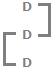Three ducks can be arranged as shown above to satisfy all the three given conditions.

QUESTION: 11

Three friends had dinner at a restaurant. When the bill was received, Amita paid 2/3 as much as Veena paid and Veena paid 1/2 as much as Tanya paid. What faction of the bill did Veena pay ?

Solution: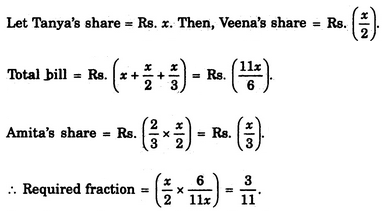QUESTION: 12

In a class, 20% of the members own only two cars each, 40% of the remaining own three cars each and the remaining members own only one car each. Which of the following statements is definitely true from the given statements ?

Solution:

Let total number of members be 100,

Then, number of members owning only 2 cars = 20.

Number of members owning 3 cars = 40% of 80 = 32.

Number of members owning only 1 car = 100 - (20 + 32) = 48.

Thus, 48% of the total members own one car each.

QUESTION: 13

When Rahul was born, his father was 32 years older than his brother and his mother was 25 years older than his sister. If Rahul's brother is 6 years older than him and his mother is 3 years younger than his father, how old was Rahul's sister when he was born ?

Solution:

When Rahul was born, his brother's age = 6 years; his father's age = (6 + 32) years = 38 years, his mother's age = (38 - 3) years = 35 years; his sister's age = (35 - 25) years = 10 years.

QUESTION: 14

A certain number of horses and an equal number of men are going somewhere. Half of the owners are on their horses' back while the remaining ones are walking along leading their horses. If the number of legs walking on the ground is 70, how many horses are there ?

Solution:

Let number of horses = number of men = x.

Then, number of legs = 4x + 2 x (x/2) = 5x.

So, 5X = 70 or x = 14.

QUESTION: 15

Ravi's brother is 3 years senior to him. His father was 28 years of age when his sister was born while his mother was 26 years of age when he was born. If his sister was 4 years of age when his brother was born, what were the ages of Ravi's father and mother respectively when his brother was born ?

Solution:

When Ravi's brother was born, let Ravi's father's age = x years and mother's age = y years.

Then, sister's age = (x - 28) years. So, x - 28 = 4 or x = 32.

Ravi's age = (y - 26) years. Age of Ravi's brother = (y - 26 + 3) years = (y - 23) years.

Now, when Ravi's brother was born, his age = 0 i.e. y - 23 = 0 or y = 23.

QUESTION: 16

The number of boys in a class is three times the number of girls. Which one of the following numbers cannot represent the total number of children in the class ?

Solution:

Let number of girls = x and number of boys = 3x.

Then, 3x + x = 4x = total number of students.

Thus, to find exact value of x, the total number of students must be divisible by 4.

QUESTION: 17

A shepherd had 17 sheep. All but nine died. How many was he left with ?

Solution:

'All but nine died' means 'All except nine died' i.e. 9 sheep remained alive.

QUESTION: 18

In a family, the father took 1/4 of the cake and he had 3 times as much as each of the other members had. The total number of family members is

Solution: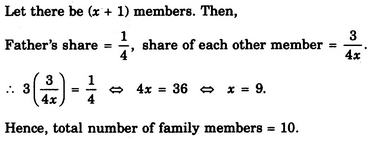QUESTION: 19

In three coloured boxes - Red, Green and Blue, 108 balls are placed. There are twice as many balls in the green and red boxes combined as there are in the blue box and twice as many in the blue box as there are in the red box. How many balls are there in the green box ?

Solution:

Let R, G and B represent the number of balls in red, green and blue boxes respectively.

Then, .

R + G + B = 108 ...(i),

G + R = 2B ...(ii)

B = 2R ...(iii)

From (ii) and (iii), we have G + R = 2x 2R = 4R or G = 3R.

Putting G = 3R and B = 2R in (i), we get:

R + 3R + 2R = 1086R = 108R = 18.

Therefore Number of balls in green box = G = 3R = (3 x 18) = 54.

QUESTION: 20

In a cricket match, five batsmen A, B, C, D and E scored an average of 36 runs. D Scored 5 more than E; E scored 8 fewer than A; B scored as many as D and E combined; and B and C scored 107 between them. How many runs did E score ?

Solution:

Total runs scored = (36 x 5) = 180.

Let the runs scored by E be x.

Then, runs scored by D = x + 5; runs scored by A = x + 8;

runs scored by B = x + x + 5 = 2x + 5;

runs scored by C = (107 - B) = 107 - (2x + 5) = 102 - 2x.

So, total runs = (x + 8) + (2x + 5) + (102 - 2x) + (x + 5) + x = 3x + 120.

Therefore 3x + 120 =1803X = 60x = 20.